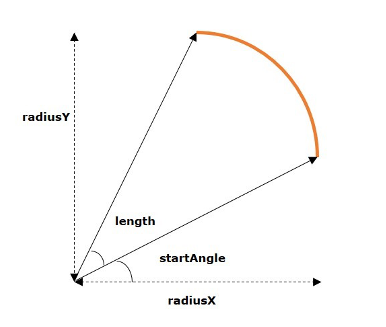# JavaFX - 2D Shapes Arc

An arc is part of a curve. It is described by the following properties −

• length − The distance along the arc.

• angle − The angle the curve makes at the centre of the circle.

• radiusX − The width of the full Ellipse of which the current arc is a part of.

• radiusY − The height of the full Ellipse of which the current arc is a part of.In JavaFX, an arc is represented by a class named Arc. This class belongs to the package javafx.scene.shape.

By instantiating this class, you can create an arc node in JavaFX.

This class has a few properties of the double datatype namely −

• centerX − The x coordinate of the center of the arc.

• centerY − The y coordinate of the center of the arc.

• radiusX − The width of the full ellipse of which the current arc is a part of.

• radiusY − The height of the full ellipse of which the current arc is a part of.

• startAngle − The starting angle of the arc in degrees.

• length − The angular extent of the arc in degrees.

To draw an arc, you need to pass values to these properties, either by passing them to the constructor of this class, in the same order, at the time of instantiation, as shown below −

```Circle circle = new Circle(centerX, centerY, radiusX, radiusY);
```

Or, by using their respective setter methods as follows −

```setCenterX(value);
setCenterY(value);